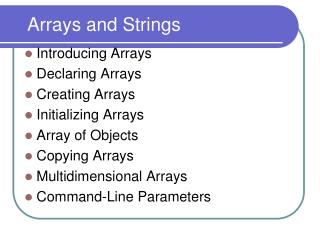DownloadDownload PresentationArrays and Strings

# Arrays and Strings

Download Presentation## Arrays and Strings

- - - - - - - - - - - - - - - - - - - - - - - - - - - E N D - - - - - - - - - - - - - - - - - - - - - - - - - - -
##### Presentation Transcript

1. Arrays and Strings • Introducing Arrays • Declaring Arrays • Creating Arrays • Initializing Arrays • Array of Objects • Copying Arrays • Multidimensional Arrays • Command-Line Parameters

2. Introducing Arrays In computer science, an array is a data structure that represents a collection of the same types of data. Java treats these arrays as objects. An Array of 10 Elementsof type double double[] myList = new double

3. Declaring Arrays • datatype[] arrayname; Example: int[] myList; • datatype arrayname[]; Example: int myList[];

4. Creating Arrays arrayName = new datatype[arraySize]; Example: myList = new double; An array is considered to be an object. Thus: myList is really a reference to 10 doubles and a field called length that contains the array’s size. For example, myList.length contains 10.

5. Declaring and Creatingin One Step datatype[] arrayname = new datatype[arraySize]; double[] myList = new double; or datatype arrayname[] = new datatype[arraySize]; double myList[] = new double;

6. Initializing Arrays • Using a loop: for (int i = 0; i < myList.length; i++) myList[i] = (double)i; • Declaring, creating, initializing in one step: double[] myList = {1.9, 2.9, 3.4, 3.5};

7. Enhanced for statement • Used to iterate through the elements of an array or collection without using a counter. • Form: for (parameter: arrayName) statement; • Parameter has two parts – a type and an identifier • ArrayName is the array through which to iterate.

8. Enhanced for Example: total = 0; for (int count=0; count < array.length; count ++) total += array [count]; Is the same as: total = 0; for(int number: array) total += number; number is actually taking on array, array, etc.

9. Using Arrays in a Gradebook • Objective: Use an array for a grade book used by a professor to store and analyze a set of student grades. • Figure 7.14 in Java book (page 317…) and Figure 7.15 on page 321.

10. Array of Objects • Declaring and creating: Circle[] circleArray = new Circle; • Initializing: for (int i=0; i<circleArray.length; i++) { circleArray[i] = new Circle(); }

11. Copying Arrays Using a loop: int[] sourceArray = {2, 3, 1, 5, 10}; int[] targetArray = new int[sourceArray.length]; for (int i = 0; i < sourceArrays.length; i++) targetArray[i] = sourceArray[i];

12. The arraycopy Utility arraycopy(sourceArray, src_pos, targetArray, tar_pos, length); Example: System.arraycopy(sourceArray, 0, targetArray, 0, sourceArray.length);

13. Multidimensional Arrays Multidimensional arrays with two dimensions are often used to represent tables of values consisting of information arranged in rows and columns called a two-dimensional array. Example: int[][] matrix = new int; or int matrix[][] = new int; for (int i=0; i<matrix.length; i++) for (int j=0; j<matrix[i].length; j++) { matrix[i][j] = (int)(Math.random()*1000); }

14. Nested array initializers A 2D array can be initialized when it is declared. Example: int b[][] = { {1, 2}, {3, 4}, {5, 6}}; creates the following array containing the values shown

15. How does Java treat a 2D array? • A 2D array is thought of as an array of arrays. • For example if b is an array with 3 rows and 2 columns, • it is considered to be a one dimensional array with 3 objects. • Each object is an array with 2 elements. b  {1, 2} b  {3, 4} b  {5, 6}

16. 2D arrays with rows of varying lengths • The way Java represents 2D arrays makes them very flexible. Lengths of rows are not required to be the same: int b[][] = {{1, 2}, {3, 4, 5, 6}}; makes b look like: b  {1, 2} b  {3, 4, 5, 6}

17. 2D Array creation examples int a[][] = new int; int a[][]; a = new int ; int c[][]; c = new int[]; //create 3 rows c = new int;//create 3 columns for row 0 C = new int;//create 2 columns for row 1 C = new int;//create 4 columns for row 2

18. length field for 2D arrays • For a 2D array declared as: int B[][]=new int; there are several “length” fields B.length contains 2, the number of rows B.length contains 3, the number of columns in row 0. B.length contains 3, the number of columns in row 1, etc.

19. Working with 2D arrays int total = 0; for (int row = 0; row < a.length; row++) { for (int column = 0; column < a[row].length; column++) total += a[row][length]; { Or for (int rows[] : a) //loop thru rows of a { //loop thru columns of the current row for (int oneValue : rows) total += oneValue; }

20. Example: • Revisit grade book using 2D arrays • Figures 7.18 and 7.19

21. Command-Line Parameters class TestMain { public static void main(String[] args) { ... } } java TestMain arg0, arg1, arg2, ..., argn

22. ProcessingCommand-Line Parameters In the main method, get the arguments from args, args, ..., args[n], which corresponds to arg0, arg1, ..., argn in the command line.

23. Example: Using Command-Line Parameters • Objective: Write a program that will perform binary operations on integers. The program receives three parameters: an operator and two integers. Java TestCommandParameters + 2 3 Java TestCommandParameters - 2 3 Java TestCommandParameters / 2 3